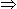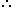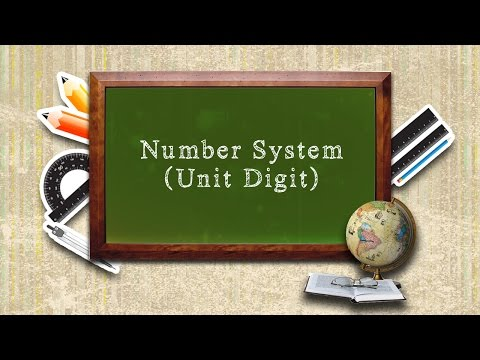Courses

# Unit Digit Calculation - Practice Test

## 5 Questions MCQ Test Quantitative Aptitude for Banking Preparation | Unit Digit Calculation - Practice Test

Description
This mock test of Unit Digit Calculation - Practice Test for UPSC helps you for every UPSC entrance exam. This contains 5 Multiple Choice Questions for UPSC Unit Digit Calculation - Practice Test (mcq) to study with solutions a complete question bank. The solved questions answers in this Unit Digit Calculation - Practice Test quiz give you a good mix of easy questions and tough questions. UPSC students definitely take this Unit Digit Calculation - Practice Test exercise for a better result in the exam. You can find other Unit Digit Calculation - Practice Test extra questions, long questions & short questions for UPSC on EduRev as well by searching above.
QUESTION: 1

### What is the unit digit in the product (365 x 659 x 771) ?

Solution:

Explanation:

Unit digit in 34 = 1Unit digit in (34)16 = 1Unit digit in 365 = Unit digit in [ (34)16 x 3 ] = (1 x 3) = 3

Unit digit in 659 = 6

Unit digit in 74Unit digit in (74)17 is 1.

Unit digit in 771 = Unit digit in [(74)17 x 73] = (1 x 3) = 3Required digit = Unit digit in (3 x 6 x 3) = 4.

QUESTION: 2

### What is the unit digit in 7105 ?

Solution:

Explanation:

Unit digit in 7105 = Unit digit in [ (74)26 x 7 ]

But, unit digit in (74)26 = 1Unit digit in 7105 = (1 x 7) = 7

QUESTION: 3

### The unit digit in the product (784 x 618 x 917 x 463) is:

Solution:

Unit digit in the given product = Unit digit in (4 x 8 x 7 x 3) = (672) = 2

QUESTION: 4

The unit digit of the sum 1289 + 2541 + 8215 + 6137 is:

Solution:

Solution: The required answer is the unit digit of the sum of the unit digits of the numbers in given sum. i.e., The unit digit of (1289 + 2541 + 8215 + 6137) = the unit digit of (9+1+5+7) = the unit digit of (22) = 2 Hence the answer is 2.

QUESTION: 5

The unit digit of the product 1022 x 729 x 889 x 971 is:

Solution:

a)2

Solution:

The required answer is the unit digit of the product of the unit digits of the numbers in given product.
i.e, The unit digit of (1022 x 729 x 889 x 971)
= the unit digit of (2 x 9 x 9 x 1)
= the unit digit of (162)
= 2

Track your progress, build streaks, highlight & save important lessons and more!

### Similar Content### Related tests